Elementary Algebra

# 1.2Use the Language of Algebra

Elementary Algebra1.2 Use the Language of Algebra

### Learning Objectives

By the end of this section, you will be able to:

• Use variables and algebraic symbols
• Simplify expressions using the order of operations
• Evaluate an expression
• Identify and combine like terms
• Translate an English phrase to an algebraic expression

### Be Prepared 1.2

A more thorough introduction to the topics covered in this section can be found in the Prealgebra chapter, The Language of Algebra.

### Use Variables and Algebraic Symbols

Suppose this year Greg is 20 years old and Alex is 23. You know that Alex is 3 years older than Greg. When Greg was 12, Alex was 15. When Greg is 35, Alex will be 38. No matter what Greg’s age is, Alex’s age will always be 3 years more, right? In the language of algebra, we say that Greg’s age and Alex’s age are variables and the 3 is a constant. The ages change (“vary”) but the 3 years between them always stays the same (“constant”). Since Greg’s age and Alex’s age will always differ by 3 years, 3 is the constant.

In algebra, we use letters of the alphabet to represent variables. So if we call Greg’s age g, then we could use $g+3g+3$ to represent Alex’s age. See Table 1.2.

Greg’s age Alex’s age
$1212$ $1515$
$2020$ $2323$
$3535$ $3838$
$gg$ $g+3g+3$
Table 1.2

The letters used to represent these changing ages are called variables. The letters most commonly used for variables are x, y, a, b, and c.

### Variable

A variable is a letter that represents a number whose value may change.

### Constant

A constant is a number whose value always stays the same.

To write algebraically, we need some operation symbols as well as numbers and variables. There are several types of symbols we will be using.

There are four basic arithmetic operations: addition, subtraction, multiplication, and division. We’ll list the symbols used to indicate these operations below ([link]). You’ll probably recognize some of them.

Operation Notation Say: The result is…
Addition $a+ba+b$ a plus b the sum of a and b
Subtraction $a−ba−b$ a minus b the difference of a and b
Multiplication $a·b,ab,(a)(b),a·b,ab,(a)(b),$ $(a)b,a(b)(a)b,a(b)$ a times b the product of a and b
Division $a÷b,a/b,ab,baa÷b,a/b,ab,ba$ a divided by b the quotient of a and b, a is called the dividend, and b is called the divisor

We perform these operations on two numbers. When translating from symbolic form to English, or from English to symbolic form, pay attention to the words “of” and “and.”

• The difference of 9 and 2 means subtract 9 and 2, in other words, 9 minus 2, which we write symbolically as $9−2.9−2.$
• The product of 4 and 8 means multiply 4 and 8, in other words 4 times 8, which we write symbolically as $4·8.4·8.$

In algebra, the cross symbol, $×,×,$ is not used to show multiplication because that symbol may cause confusion. Does 3xy mean $3×y3×y$ (‘three times y’) or $3·x·y3·x·y$ (three times x times y)? To make it clear, use $··$ or parentheses for multiplication.

When two quantities have the same value, we say they are equal and connect them with an equal sign.

### Equality Symbol

$a=ba=b$ is read “a is equal to b

The symbol $“=”“=”$ is called the equal sign.

On the number line, the numbers get larger as they go from left to right. The number line can be used to explain the symbols “<” and “>.”

### Inequality

$a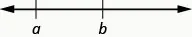$a>bis read “ais greater thanb”ais to the right ofbon the number linea>bis read “ais greater thanb”ais to the right ofbon the number line$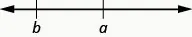The expressions a < b or a > b can be read from left to right or right to left, though in English we usually read from left to right (Table 1.3). In general, a < b is equivalent to b > a. For example 7 < 11 is equivalent to 11 > 7. And a > b is equivalent to b < a. For example 17 > 4 is equivalent to 4 < 17.

Inequality Symbols Words
$a≠ba≠b$ a is not equal to b
a < b a is less than b
$a≤ba≤b$ a is less than or equal to b
a > b a is greater than b
$a≥ba≥b$ a is greater than or equal to b
Table 1.3

### Example 1.12

Translate from algebra into English:

$17≤2617≤26$ $8≠17−38≠17−3$ $12>27÷312>27÷3$ $y+7<19y+7<19$

### Try It 1.23

Translate from algebra into English:

$14≤2714≤27$ $19−2≠819−2≠8$ $12>4÷212>4÷2$ $x−7<1x−7<1$

### Try It 1.24

Translate from algebra into English:

$19≥1519≥15$ $7=12−57=12−5$ $15÷3<815÷3<8$ $y+3>6y+3>6$

Grouping symbols in algebra are much like the commas, colons, and other punctuation marks in English. They help to make clear which expressions are to be kept together and separate from other expressions. We will introduce three types now.

### Grouping Symbols

$Parentheses()Brackets[]Braces{}Parentheses()Brackets[]Braces{}$

Here are some examples of expressions that include grouping symbols. We will simplify expressions like these later in this section.

$8(14−8)21−3[2+4(9−8)]24÷{13−2[1(6−5)+4]}8(14−8)21−3[2+4(9−8)]24÷{13−2[1(6−5)+4]}$

What is the difference in English between a phrase and a sentence? A phrase expresses a single thought that is incomplete by itself, but a sentence makes a complete statement. “Running very fast” is a phrase, but “The football player was running very fast” is a sentence. A sentence has a subject and a verb. In algebra, we have expressions and equations.

### Expression

An expression is a number, a variable, or a combination of numbers and variables using operation symbols.

An expression is like an English phrase. Here are some examples of expressions:

Expression Words English Phrase
$3+53+5$ 3 plus 5 the sum of three and five
$n−1n−1$ n minus one the difference of n and one
$6·76·7$ 6 times 7 the product of six and seven
$xyxy$ x divided by y the quotient of x and y

Notice that the English phrases do not form a complete sentence because the phrase does not have a verb.

An equation is two expressions linked with an equal sign. When you read the words the symbols represent in an equation, you have a complete sentence in English. The equal sign gives the verb.

### Equation

An equation is two expressions connected by an equal sign.

Here are some examples of equations.

Equation English Sentence
$3+5=83+5=8$ The sum of three and five is equal to eight.
$n−1=14n−1=14$ n minus one equals fourteen.
$6·7=426·7=42$ The product of six and seven is equal to forty-two.
$x=53x=53$ x is equal to fifty-three.
$y+9=2y−3y+9=2y−3$ y plus nine is equal to two y minus three.

### Example 1.13

Determine if each is an expression or an equation:

$2(x+3)=102(x+3)=10$ $4(y−1)+14(y−1)+1$ $x÷25x÷25$ $y+8=40y+8=40$

### Try It 1.25

Determine if each is an expression or an equation: $3(x−7)=273(x−7)=27$ $5(4y−2)−75(4y−2)−7$.

### Try It 1.26

Determine if each is an expression or an equation: $y3÷14y3÷14$ $4x−6=224x−6=22$.

Suppose we need to multiply 2 nine times. We could write this as $2·2·2·2·2·2·2·2·2.2·2·2·2·2·2·2·2·2.$ This is tedious and it can be hard to keep track of all those 2s, so we use exponents. We write $2·2·22·2·2$ as $2323$ and $2·2·2·2·2·2·2·2·22·2·2·2·2·2·2·2·2$ as $29.29.$ In expressions such as $23,23,$ the 2 is called the base and the 3 is called the exponent. The exponent tells us how many times we need to multiply the base.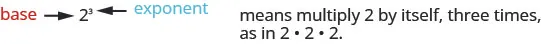We read $2323$ as “two to the third power” or “two cubed.”

We say $2323$ is in exponential notation and $2·2·22·2·2$ is in expanded notation.

### Exponential Notation

$anan$ means multiply a by itself, n times.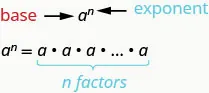The expression $anan$ is read a to the $nthnth$ power.

While we read $anan$ as “a to the $nthnth$ power,” we usually read:

• $a2a2$a squared”
• $a3a3$a cubed”

We’ll see later why $a2a2$ and $a3a3$ have special names.

Table 1.4 shows how we read some expressions with exponents.

Expression In Words
$7272$ 7 to the second power or 7 squared
$5353$ 5 to the third power or 5 cubed
$9494$ 9 to the fourth power
$125125$ $1212$ to the fifth power
Table 1.4

### Example 1.14

Simplify: $34.34.$

### Try It 1.27

Simplify: $5353$ $17.17.$

### Try It 1.28

Simplify: $7272$ $05.05.$

### Simplify Expressions Using the Order of Operations

To simplify an expression means to do all the math possible. For example, to simplify $4·2+14·2+1$ we’d first multiply $4·24·2$ to get 8 and then add the 1 to get 9. A good habit to develop is to work down the page, writing each step of the process below the previous step. The example just described would look like this:

$4·2+18+194·2+18+19$

By not using an equal sign when you simplify an expression, you may avoid confusing expressions with equations.

### Simplify an Expression

To simplify an expression, do all operations in the expression.

We’ve introduced most of the symbols and notation used in algebra, but now we need to clarify the order of operations. Otherwise, expressions may have different meanings, and they may result in different values. For example, consider the expression:

$4+3·74+3·7$

If you simplify this expression, what do you get?

Some students say 49,

$4+3·7Since4+3gives7.7·7And7·7is49.494+3·7Since4+3gives7.7·7And7·7is49.49$

Others say 25,

$4+3·7Since3·7is21.4+21And21+4makes25.254+3·7Since3·7is21.4+21And21+4makes25.25$

Imagine the confusion in our banking system if every problem had several different correct answers!

The same expression should give the same result. So mathematicians early on established some guidelines that are called the Order of Operations.

### How To

#### Perform the Order of Operations.

1. Step 1.
Parentheses and Other Grouping Symbols
• Simplify all expressions inside the parentheses or other grouping symbols, working on the innermost parentheses first.
2. Step 2.
Exponents
• Simplify all expressions with exponents.
3. Step 3.
Multiplication and Division
• Perform all multiplication and division in order from left to right. These operations have equal priority.
4. Step 4.
• Perform all addition and subtraction in order from left to right. These operations have equal priority.

### Manipulative Mathematics

Doing the Manipulative Mathematics activity “Game of 24” give you practice using the order of operations.

Students often ask, “How will I remember the order?” Here is a way to help you remember: Take the first letter of each key word and substitute the silly phrase: “Please Excuse My Dear Aunt Sally.”

$ParenthesesPleaseExponentsExcuseMultiplicationDivisionMyDearAdditionSubtractionAuntSallyParenthesesPleaseExponentsExcuseMultiplicationDivisionMyDearAdditionSubtractionAuntSally$

It’s good that “My Dear” goes together, as this reminds us that multiplication and division have equal priority. We do not always do multiplication before division or always do division before multiplication. We do them in order from left to right.

Similarly, “Aunt Sally” goes together and so reminds us that addition and subtraction also have equal priority and we do them in order from left to right.

Let’s try an example.

### Example 1.15

Simplify: $4+3·74+3·7$ $(4+3)·7.(4+3)·7.$

### Try It 1.29

Simplify: $12−5·212−5·2$ $(12−5)·2.(12−5)·2.$

### Try It 1.30

Simplify: $8+3·98+3·9$ $(8+3)·9.(8+3)·9.$

### Example 1.16

Simplify: $18÷6+4(5−2).18÷6+4(5−2).$

### Try It 1.31

Simplify: $30÷5+10(3−2).30÷5+10(3−2).$

### Try It 1.32

Simplify: $70÷10+4(6−2).70÷10+4(6−2).$

When there are multiple grouping symbols, we simplify the innermost parentheses first and work outward.

### Example 1.17

Simplify: $5+23+3[6−3(4−2)].5+23+3[6−3(4−2)].$

### Try It 1.33

Simplify: $9+53−[4(9+3)].9+53−[4(9+3)].$

### Try It 1.34

Simplify: $72−2[4(5+1)].72−2[4(5+1)].$

### Evaluate an Expression

In the last few examples, we simplified expressions using the order of operations. Now we’ll evaluate some expressions—again following the order of operations. To evaluate an expression means to find the value of the expression when the variable is replaced by a given number.

### Evaluate an Expression

To evaluate an expression means to find the value of the expression when the variable is replaced by a given number.

To evaluate an expression, substitute that number for the variable in the expression and then simplify the expression.

### Example 1.18

Evaluate $7x−4,7x−4,$ when $x=5x=5$ and $x=1.x=1.$

### Try It 1.35

Evaluate $8x−3,8x−3,$ when $x=2x=2$ and $x=1.x=1.$

### Try It 1.36

Evaluate $4y−4,4y−4,$ when $y=3y=3$ and $y=5.y=5.$

### Example 1.19

Evaluate $x=4,x=4,$ when $x2x2$ $3x.3x.$

### Try It 1.37

Evaluate $x=3,x=3,$ when $x2x2$ $4x.4x.$

### Try It 1.38

Evaluate $x=6,x=6,$ when $x3x3$ $2x.2x.$

### Example 1.20

Evaluate $2x2+3x+82x2+3x+8$ when $x=4.x=4.$

### Try It 1.39

Evaluate $3x2+4x+13x2+4x+1$ when $x=3.x=3.$

### Try It 1.40

Evaluate $6x2−4x−76x2−4x−7$ when $x=2.x=2.$

### Identify and Combine Like Terms

Algebraic expressions are made up of terms. A term is a constant, or the product of a constant and one or more variables.

### Term

A term is a constant, or the product of a constant and one or more variables.

Examples of terms are $7,y,5x2,9a,andb5.7,y,5x2,9a,andb5.$

The constant that multiplies the variable is called the coefficient.

### Coefficient

The coefficient of a term is the constant that multiplies the variable in a term.

Think of the coefficient as the number in front of the variable. The coefficient of the term 3x is 3. When we write x, the coefficient is 1, since $x=1·x.x=1·x.$

### Example 1.21

Identify the coefficient of each term: 14y $15x215x2$ a.

### Try It 1.41

Identify the coefficient of each term: $17x17x$ $41b241b2$ z.

### Try It 1.42

Identify the coefficient of each term: 9p $13a313a3$ $y3.y3.$

Some terms share common traits. Look at the following 6 terms. Which ones seem to have traits in common?

$5x7n243x9n25x7n243x9n2$

The 7 and the 4 are both constant terms.

The 5x and the 3x are both terms with x.

The $n2n2$ and the $9n29n2$ are both terms with $n2.n2.$

When two terms are constants or have the same variable and exponent, we say they are like terms.

• 7 and 4 are like terms.
• 5x and 3x are like terms.
• $x2x2$ and $9x29x2$ are like terms.

### Like Terms

Terms that are either constants or have the same variables raised to the same powers are called like terms.

### Example 1.22

Identify the like terms: $y3,y3,$ $7x2,7x2,$ 14, 23, $4y3,4y3,$ 9x, $5x2.5x2.$

### Try It 1.43

Identify the like terms: $9,9,$ $2x3,2x3,$ $y2,y2,$ $8x3,8x3,$ $15,15,$ $9y,9y,$ $11y2.11y2.$

### Try It 1.44

Identify the like terms: $4x3,4x3,$ $8x2,8x2,$ 19, $3x2,3x2,$ 24, $6x3.6x3.$

Adding or subtracting terms forms an expression. In the expression $2x2+3x+8,2x2+3x+8,$ from Example 1.20, the three terms are $2x2,3x,2x2,3x,$ and 8.

### Example 1.23

Identify the terms in each expression.

1. $9x2+7x+129x2+7x+12$
2. $8x+3y8x+3y$

### Try It 1.45

Identify the terms in the expression $4x2+5x+17.4x2+5x+17.$

### Try It 1.46

Identify the terms in the expression $5x+2y.5x+2y.$

If there are like terms in an expression, you can simplify the expression by combining the like terms. What do you think $4x+7x+x4x+7x+x$ would simplify to? If you thought 12x, you would be right!

$4x+7x+xx+x+x+x+x+x+x+x+x+x+x+x12x4x+7x+xx+x+x+x+x+x+x+x+x+x+x+x12x$

Add the coefficients and keep the same variable. It doesn’t matter what x is—if you have 4 of something and add 7 more of the same thing and then add 1 more, the result is 12 of them. For example, 4 oranges plus 7 oranges plus 1 orange is 12 oranges. We will discuss the mathematical properties behind this later.

Simplify: $4x+7x+x.4x+7x+x.$

### Example 1.24

#### How To Combine Like Terms

Simplify: $2x2+3x+7+x2+4x+5.2x2+3x+7+x2+4x+5.$

### Try It 1.47

Simplify: $3x2+7x+9+7x2+9x+8.3x2+7x+9+7x2+9x+8.$

### Try It 1.48

Simplify: $4y2+5y+2+8y2+4y+5.4y2+5y+2+8y2+4y+5.$

### How To

#### Combine Like Terms.

1. Step 1. Identify like terms.
2. Step 2. Rearrange the expression so like terms are together.
3. Step 3. Add or subtract the coefficients and keep the same variable for each group of like terms.

### Translate an English Phrase to an Algebraic Expression

In the last section, we listed many operation symbols that are used in algebra, then we translated expressions and equations into English phrases and sentences. Now we’ll reverse the process. We’ll translate English phrases into algebraic expressions. The symbols and variables we’ve talked about will help us do that. Table 1.5 summarizes them.

Operation Phrase Expression
the sum of $aa$ and b
$aa$ increased by b
b more than $aa$
the total of $aa$ and b
b added to $aa$
$a+ba+b$
Subtraction $aa$ minus b
the difference of $aa$ and b
$aa$ decreased by b
b less than $aa$
b subtracted from $aa$
$a−ba−b$
Multiplication $aa$ times b
the product of $aa$ and b
twice $aa$
$a·b,ab,a(b),(a)(b)a·b,ab,a(b),(a)(b)$

2a
Division $aa$ divided by b
the quotient of $aa$ and b
the ratio of $aa$ and b
b divided into $aa$
$a÷b,a/b,ab,baa÷b,a/b,ab,ba$
Table 1.5

Look closely at these phrases using the four operations: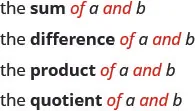Each phrase tells us to operate on two numbers. Look for the words of and and to find the numbers.

### Example 1.25

Translate each English phrase into an algebraic expression: the difference of $17x17x$ and $55$ the quotient of $10x210x2$ and $7.7.$

### Try It 1.49

Translate the English phrase into an algebraic expression: the difference of $14x214x2$ and 13 the quotient of 12x and 2.

### Try It 1.50

Translate the English phrase into an algebraic expression: the sum of $17y217y2$ and $1919$ the product of $77$ and $y.y.$

How old will you be in eight years? What age is eight more years than your age now? Did you add 8 to your present age? Eight “more than” means 8 added to your present age. How old were you seven years ago? This is 7 years less than your age now. You subtract 7 from your present age. Seven “less than” means 7 subtracted from your present age.

### Example 1.26

Translate the English phrase into an algebraic expression: Seventeen more than y Nine less than $9x2.9x2.$

### Try It 1.51

Translate the English phrase into an algebraic expression: Eleven more than $xx$ Fourteen less than $11a.11a.$

### Try It 1.52

Translate the English phrase into an algebraic expression: 13 more than z 18 less than 8x.

### Example 1.27

Translate the English phrase into an algebraic expression: five times the sum of m and n the sum of five times m and n.

### Try It 1.53

Translate the English phrase into an algebraic expression: four times the sum of p and q the sum of four times p and q.

### Try It 1.54

Translate the English phrase into an algebraic expression: the difference of two times x and 8, two times the difference of x and 8.

Later in this course, we’ll apply our skills in algebra to solving applications. The first step will be to translate an English phrase to an algebraic expression. We’ll see how to do this in the next two examples.

### Example 1.28

The length of a rectangle is 6 less than the width. Let w represent the width of the rectangle. Write an expression for the length of the rectangle.

### Try It 1.55

The length of a rectangle is 7 less than the width. Let w represent the width of the rectangle. Write an expression for the length of the rectangle.

### Try It 1.56

The width of a rectangle is 6 less than the length. Let l represent the length of the rectangle. Write an expression for the width of the rectangle.

### Example 1.29

June has dimes and quarters in her purse. The number of dimes is three less than four times the number of quarters. Let q represent the number of quarters. Write an expression for the number of dimes.

### Try It 1.57

Geoffrey has dimes and quarters in his pocket. The number of dimes is eight less than four times the number of quarters. Let q represent the number of quarters. Write an expression for the number of dimes.

### Try It 1.58

Lauren has dimes and nickels in her purse. The number of dimes is three more than seven times the number of nickels. Let n represent the number of nickels. Write an expression for the number of dimes.

### Section 1.2 Exercises

#### Practice Makes Perfect

Use Variables and Algebraic Symbols

In the following exercises, translate from algebra to English.

83.

$16 − 9 16 − 9$

84.

$3 · 9 3 · 9$

85.

$28 ÷ 4 28 ÷ 4$

86.

$x + 11 x + 11$

87.

$( 2 ) ( 7 ) ( 2 ) ( 7 )$

88.

$( 4 ) ( 8 ) ( 4 ) ( 8 )$

89.

$14 < 21 14 < 21$

90.

$17 < 35 17 < 35$

91.

$36 ≥ 19 36 ≥ 19$

92.

$6 n = 36 6 n = 36$

93.

$y − 1 > 6 y − 1 > 6$

94.

$y − 4 > 8 y − 4 > 8$

95.

$2 ≤ 18 ÷ 6 2 ≤ 18 ÷ 6$

96.

$a ≠ 1 · 12 a ≠ 1 · 12$

In the following exercises, determine if each is an expression or an equation.

97.

$9 · 6 = 54 9 · 6 = 54$

98.

$7 · 9 = 63 7 · 9 = 63$

99.

$5 · 4 + 3 5 · 4 + 3$

100.

$x + 7 x + 7$

101.

$x + 9 x + 9$

102.

$y − 5 = 25 y − 5 = 25$

Simplify Expressions Using the Order of Operations

In the following exercises, simplify each expression.

103.

$5 3 5 3$

104.

$8 3 8 3$

105.

$2 8 2 8$

106.

$10 5 10 5$

In the following exercises, simplify using the order of operations.

107.

$3+8·53+8·5$ $(3+8)·5(3+8)·5$

108.

$2+6·32+6·3$ $(2+6)·3(2+6)·3$

109.

$2 3 − 12 ÷ ( 9 − 5 ) 2 3 − 12 ÷ ( 9 − 5 )$

110.

$3 2 − 18 ÷ ( 11 − 5 ) 3 2 − 18 ÷ ( 11 − 5 )$

111.

$3 · 8 + 5 · 2 3 · 8 + 5 · 2$

112.

$4 · 7 + 3 · 5 4 · 7 + 3 · 5$

113.

$2 + 8 ( 6 + 1 ) 2 + 8 ( 6 + 1 )$

114.

$4 + 6 ( 3 + 6 ) 4 + 6 ( 3 + 6 )$

115.

$4 · 12 / 8 4 · 12 / 8$

116.

$2 · 36 / 6 2 · 36 / 6$

117.

$( 6 + 10 ) ÷ ( 2 + 2 ) ( 6 + 10 ) ÷ ( 2 + 2 )$

118.

$( 9 + 12 ) ÷ ( 3 + 4 ) ( 9 + 12 ) ÷ ( 3 + 4 )$

119.

$20 ÷ 4 + 6 · 5 20 ÷ 4 + 6 · 5$

120.

$33 ÷ 3 + 8 · 2 33 ÷ 3 + 8 · 2$

121.

$3 2 + 7 2 3 2 + 7 2$

122.

$( 3 + 7 ) 2 ( 3 + 7 ) 2$

123.

$3 ( 1 + 9 · 6 ) − 4 2 3 ( 1 + 9 · 6 ) − 4 2$

124.

$5 ( 2 + 8 · 4 ) − 7 2 5 ( 2 + 8 · 4 ) − 7 2$

125.

$2 [ 1 + 3 ( 10 − 2 ) ] 2 [ 1 + 3 ( 10 − 2 ) ]$

126.

$5 [ 2 + 4 ( 3 − 2 ) ] 5 [ 2 + 4 ( 3 − 2 ) ]$

Evaluate an Expression

In the following exercises, evaluate the following expressions.

127.

$7x+87x+8$ when $x=2x=2$

128.

$8x−68x−6$ when $x=7x=7$

129.

$x2x2$ when $x=12x=12$

130.

$x3x3$ when $x=5x=5$

131.

$x5x5$ when $x=2x=2$

132.

$4x4x$ when $x=2x=2$

133.

$x2+3x−7x2+3x−7$ when $x=4x=4$

134.

$6x+3y−96x+3y−9$ when
$x=6,y=9x=6,y=9$

135.

$(x−y)2(x−y)2$ when
$x=10,y=7x=10,y=7$

136.

$(x+y)2(x+y)2$ when $x=6,y=9x=6,y=9$

137.

$a2+b2a2+b2$ when $a=3,b=8a=3,b=8$

138.

$r2−s2r2−s2$ when $r=12,s=5r=12,s=5$

139.

$2l+2w2l+2w$ when
$l=15,w=12l=15,w=12$

140.

$2l+2w2l+2w$ when
$l=18,w=14l=18,w=14$

Simplify Expressions by Combining Like Terms

In the following exercises, identify the coefficient of each term.

141.

8a

142.

13m

143.

$5 r 2 5 r 2$

144.

$6 x 3 6 x 3$

In the following exercises, identify the like terms.

145.

$x 3 , 8 x , 14 , 8 y , 5 , 8 x 3 x 3 , 8 x , 14 , 8 y , 5 , 8 x 3$

146.

$6 z , 3 w 2 , 1 , 6 z 2 , 4 z , w 2 6 z , 3 w 2 , 1 , 6 z 2 , 4 z , w 2$

147.

$9 a , a 2 , 16 , 16 b 2 , 4 , 9 b 2 9 a , a 2 , 16 , 16 b 2 , 4 , 9 b 2$

148.

$3 , 25 r 2 , 10 s , 10 r , 4 r 2 , 3 s 3 , 25 r 2 , 10 s , 10 r , 4 r 2 , 3 s$

In the following exercises, identify the terms in each expression.

149.

$15 x 2 + 6 x + 2 15 x 2 + 6 x + 2$

150.

$11 x 2 + 8 x + 5 11 x 2 + 8 x + 5$

151.

$10 y 3 + y + 2 10 y 3 + y + 2$

152.

$9 y 3 + y + 5 9 y 3 + y + 5$

In the following exercises, simplify the following expressions by combining like terms.

153.

$10 x + 3 x 10 x + 3 x$

154.

$15 x + 4 x 15 x + 4 x$

155.

$4 c + 2 c + c 4 c + 2 c + c$

156.

$6 y + 4 y + y 6 y + 4 y + y$

157.

$7 u + 2 + 3 u + 1 7 u + 2 + 3 u + 1$

158.

$8 d + 6 + 2 d + 5 8 d + 6 + 2 d + 5$

159.

$10 a + 7 + 5 a − 2 + 7 a − 4 10 a + 7 + 5 a − 2 + 7 a − 4$

160.

$7 c + 4 + 6 c − 3 + 9 c − 1 7 c + 4 + 6 c − 3 + 9 c − 1$

161.

$3 x 2 + 12 x + 11 + 14 x 2 + 8 x + 5 3 x 2 + 12 x + 11 + 14 x 2 + 8 x + 5$

162.

$5 b 2 + 9 b + 10 + 2 b 2 + 3 b − 4 5 b 2 + 9 b + 10 + 2 b 2 + 3 b − 4$

Translate an English Phrase to an Algebraic Expression

In the following exercises, translate the phrases into algebraic expressions.

163.

the difference of 14 and 9

164.

the difference of 19 and 8

165.

the product of 9 and 7

166.

the product of 8 and 7

167.

the quotient of 36 and 9

168.

the quotient of 42 and 7

169.

the sum of 8x and 3x

170.

the sum of 13x and 3x

171.

the quotient of y and 3

172.

the quotient of y and 8

173.

eight times the difference of y and nine

174.

seven times the difference of y and one

175.

Eric has rock and classical CDs in his car. The number of rock CDs is 3 more than the number of classical CDs. Let c represent the number of classical CDs. Write an expression for the number of rock CDs.

176.

The number of girls in a second-grade class is 4 less than the number of boys. Let b represent the number of boys. Write an expression for the number of girls.

177.

Greg has nickels and pennies in his pocket. The number of pennies is seven less than twice the number of nickels. Let $nn$ represent the number of nickels. Write an expression for the number of pennies.

178.

Jeannette has $5 and$10 bills in her wallet. The number of fives is three more than six times the number of tens. Let t represent the number of tens. Write an expression for the number of fives.

#### Everyday Math

179.

Car insurance Justin’s car insurance has a $750 deductible per incident. This means that he pays$750 and his insurance company will pay all costs beyond $750. If Justin files a claim for$2,100.

1. how much will he pay?
2. how much will his insurance company pay?
180.

Home insurance Armando’s home insurance has a $2,500 deductible per incident. This means that he pays$2,500 and the insurance company will pay all costs beyond $2,500. If Armando files a claim for$19,400.

1. how much will he pay?
2. how much will the insurance company pay?

#### Writing Exercises

181.

Explain the difference between an expression and an equation.

182.

Why is it important to use the order of operations to simplify an expression?

183.

Explain how you identify the like terms in the expression $8a2+4a+9−a2−1.8a2+4a+9−a2−1.$

184.

Explain the difference between the phrases “4 times the sum of x and y” and “the sum of 4 times x and y.”

#### Self Check

Use this checklist to evaluate your mastery of the objectives of this section.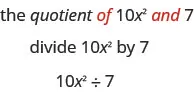After reviewing this checklist, what will you do to become confident for all objectives?

Order a print copy

As an Amazon Associate we earn from qualifying purchases.